## Knowing maths, knowing me

• In this activity students find out facts about each other but through doing maths:

1 students write maths exercises and upload them on the project twinspace

2 students from partner schools solve maths problems and find out e.g how old someone is

3 students write about students from partner school and upload their solutions (reports, ID cards...)

Example:

Find the product of the quotient of numbers 54 and 9 and the difference of numbers 14 and 12.You'll get my age.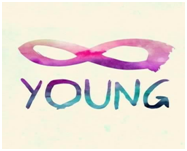Find the quotient of the product of numbers 11 and 2 and the sum of numbers 8 and 3.You'll get my house number.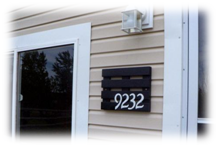Find the sum  of the difference of numbers 16 and 3 and the product of numbers 6 and 4.You'll get my shoe size.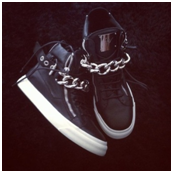Find the quotient of the sum of numbers 6 and 3 and the difference of numbers 21 and 18.You'll get the number of my kittens.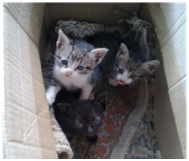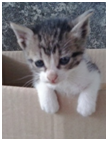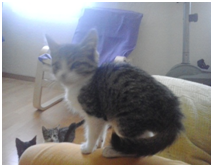Find the quotient of the product of numbers 255 and 3 and the sum of numbers 2 and 3.You'll get my height in centimeters.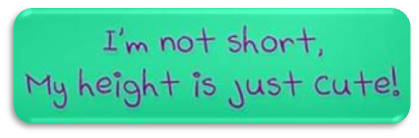How to use this in a lesson:

1 Start your lesson with a running dictation to repeat numbers or maths vocabulary.

2 Before you give your students to calculate maths problems from their eTwinning partners, calculate them yourself and some of the numbers on the board. Ask your students what they think these numbers represent (age/height/kilometres/house number/shoe size). Let your students discuss their predictions with arguments and use vocabulary to express their opinion/agreement/disagreement.

I agree / don't agree / I disagree...because

Why do you think that? / I think... / don't think...

In my opinion...

3 Give your students maths problems and let them calculate individually or in pairs.

4 When they have finished the calculations, check them and discuss the numbers  written on the board to check the predictions they had made in the 2nd step of the lesson. Your students can use expressions like: you were right and I was wrong / we were both right/wrong.

5 Now that your students have calculated information about their eTwinning partners, they can write sentences about them e.g. Ena is 15 years old. She is in class 8. Her house number is 54. Her granny is 83 years old. etc.

You can do this task at school or give as homework and when your students have written the sentences they upload them in the Twinspace in a word documment, as a comment or in any other way that suits you and your partners.